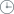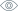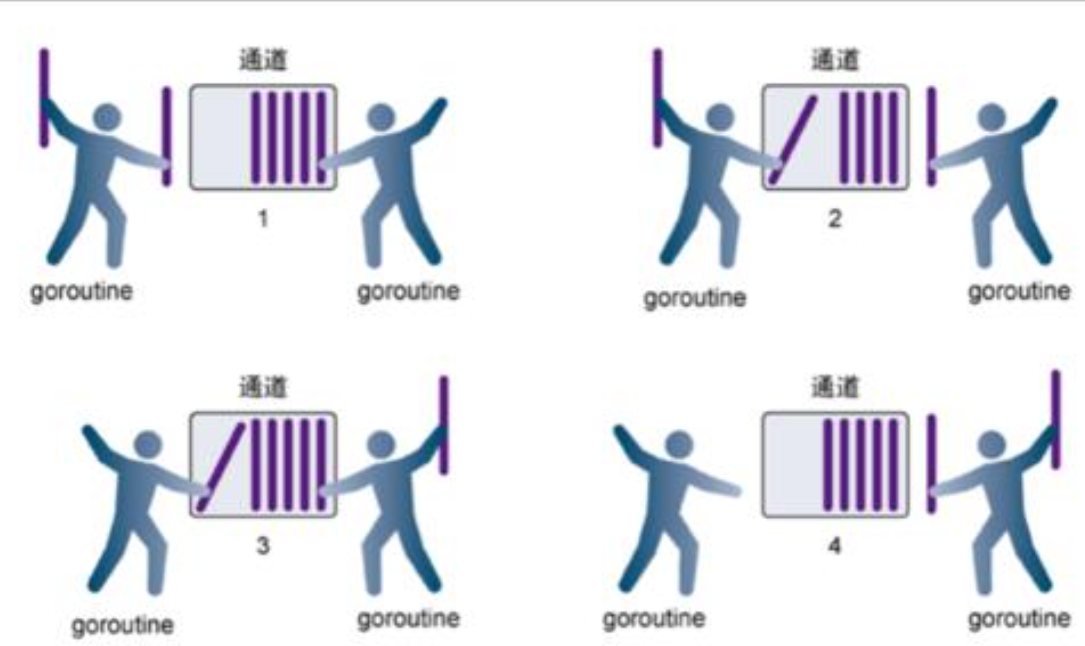### 关于Go channel 简单说明2021.01.051066channel可以与Unix shell 中的双向管道做类比：可以通过它发送或者接收值。

ci := make(chan int)
cs := make(chan string)
cf := make(chan interface{})

channel通过操作符<-来接收和发送数据

ch <- v    // 发送v到channel ch.
v := <-ch  // 从ch中接收数据，并赋值给v

func sum(a []int, c chan int) {
total := 0
for _, v := range a {
total += v
}
c <- total //将total发送到c中...
}

a3 := []int{1, 2, 3, 3, 2, 1}
c3 := make(chan int)
go sum(a3[:len(a3)/2], c3)
go sum(a3[len(a3)/2:], c3)
x, y := <-c3, <-c3 //从C中获取
fmt.Println(x, y, x+y)

Go也允许指定channel的缓冲大小，很简单，就是channel可以存储多少元素。ch:= make(chan bool, 4)，

c := make(chan int, 2)//修改2为1就报错，修改2为3可以正常运行
c <- 1
c <- 2
fmt.Println(<-c)
fmt.Println(<-c)

Range和Close
func fibonacci(n int, c chan int) {
x, y := 1, 1
for i := 0; i < n; i++ {
c <- x
x, y = y, x + y
}
close(c)
}

func main() {
c := make(chan int, 10)
go fibonacci(cap(c), c)
for i := range c {
fmt.Println(i)
}
}

for i := range c能够不断的读取channel里面的数据，直到该channel被显式的关闭。

channel可以与Unix shell 中的双向管道做类比：可以通过它发送或者接收值。

ci := make(chan int)
cs := make(chan string)
cf := make(chan interface{})

channel通过操作符<-来接收和发送数据

ch <- v    // 发送v到channel ch.
v := <-ch  // 从ch中接收数据，并赋值给v

func sum(a []int, c chan int) {
total := 0
for _, v := range a {
total += v
}
c <- total //将total发送到c中...
}

a3 := []int{1, 2, 3, 3, 2, 1}
c3 := make(chan int)
go sum(a3[:len(a3)/2], c3)
go sum(a3[len(a3)/2:], c3)
x, y := <-c3, <-c3 //从C中获取
fmt.Println(x, y, x+y)

Go也允许指定channel的缓冲大小，很简单，就是channel可以存储多少元素。ch:= make(chan bool, 4)，

c := make(chan int, 2)//修改2为1就报错，修改2为3可以正常运行
c <- 1
c <- 2
fmt.Println(<-c)
fmt.Println(<-c)

Range和Close
func fibonacci(n int, c chan int) {
x, y := 1, 1
for i := 0; i < n; i++ {
c <- x
x, y = y, x + y
}
close(c)
}

func main() {
c := make(chan int, 10)
go fibonacci(cap(c), c)
for i := range c {
fmt.Println(i)
}
}

for i := range c能够不断的读取channel里面的数据，直到该channel被显式的关闭。

### 相关新闻##### 微信开发-微信企业号2015.11.263359##### 301的意义2015.10.222018##### 成都微信开发-你们的密码安全吗2015.09.162733